Linked to an optional connector or a Calculation node, the Parameter node defines the minimum and maximum values, and the number of decimal places for these values. The output value is set using a slider.

To add a Parameter node, right-click in the Graph Editor and select Create Node  > Calculation  > Formula.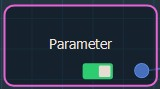Double click on the node to open its parameters: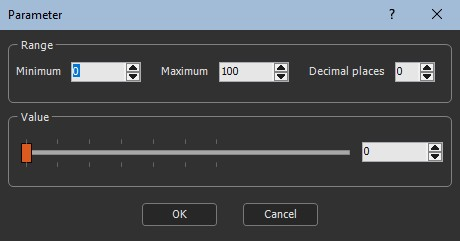### Editing a Parameter node

The parameters window allows you to set the Range of the output value, and then you can use the slider to set an exact value.

• Enter the Minimum and Maximum values, number of decimal places for these values.
• Use the slider to set the output Value.

The Parameter node has one output connector that links to a Calculation node or an optional connector.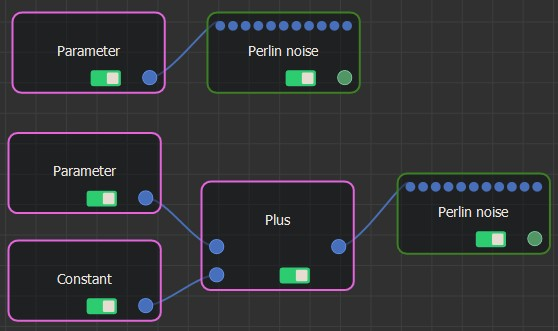### Example

In the example below, we use two Parameter nodes to drive the terrain size of the Perlin noise generator and the maximum height of the Min & max heights terrain transformer.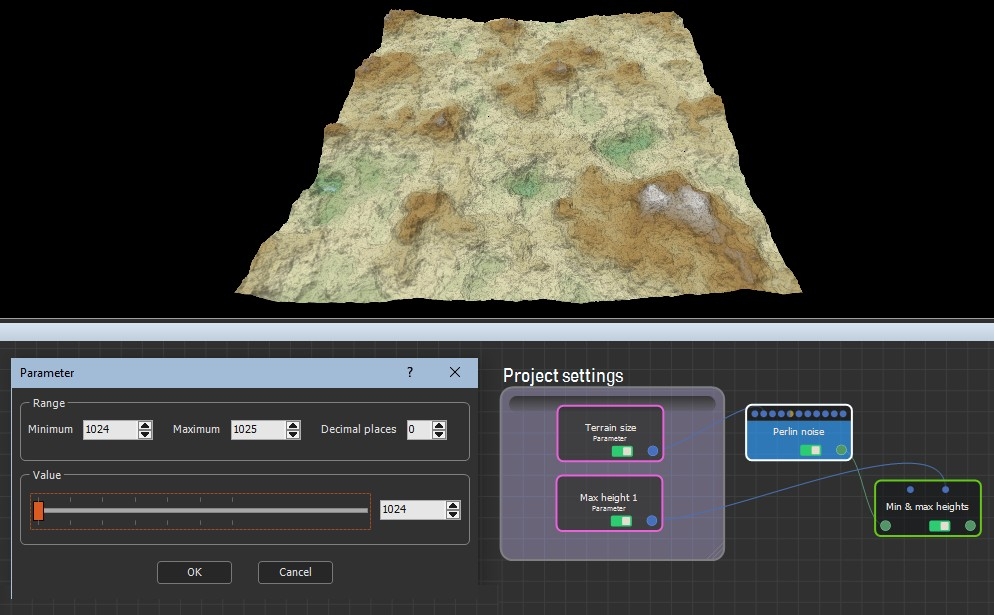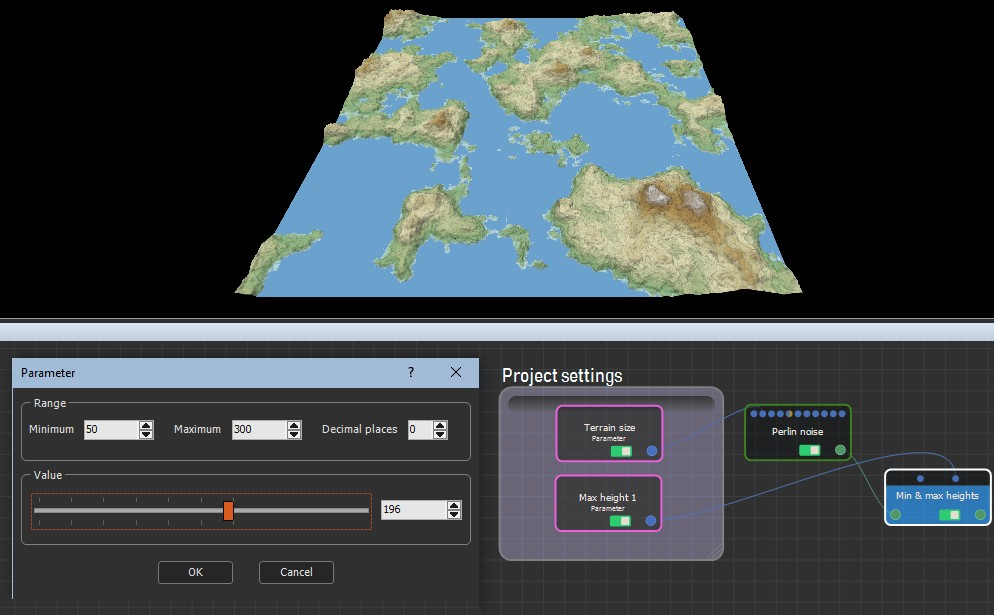### Parameters

Parameter Use
Minimum Sets a minimum output value
Maximum Sets a maximum output value
Value Defines the exact output value